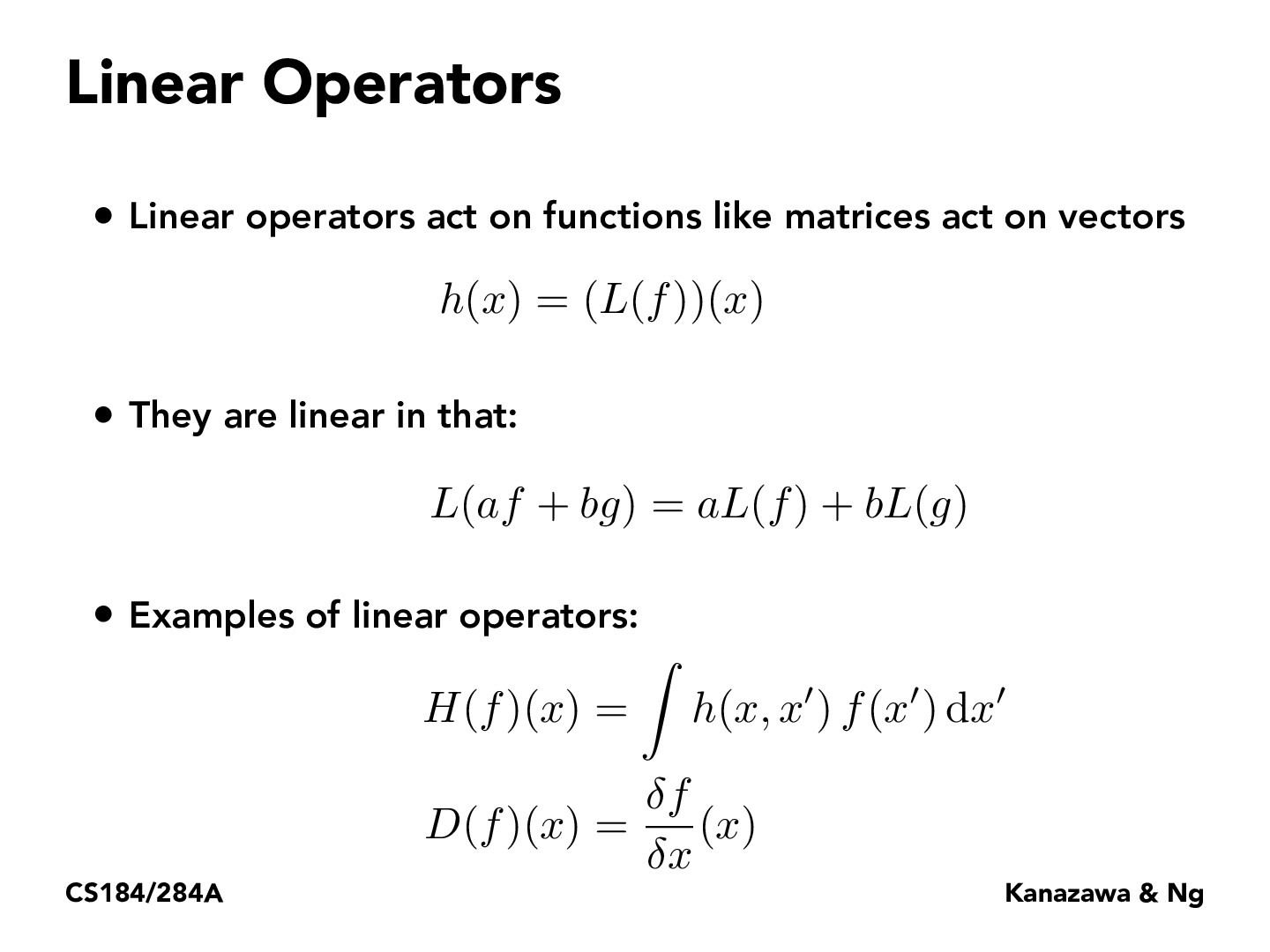Lecture 13: Global Illumination & Path Tracing (34)philippe-eecs

Linear operations act on functions, but does it work like linear mappings in linear Algebra? There is a theorem where all linear functions can be represented as a matrix transformation of some sort. Does something of this sort apply to Linear operations? Can we break down the operation into some sort of matrix application on a function?Leon-Shao

@philippe-eecsI think linear operator can be represented as matrix form but there are various types of linear operators, as in the examples of this slide we got partial derivative and integration as well. To me I think it's just not that useful for a common form for these different types.

You must be enrolled in the course to comment